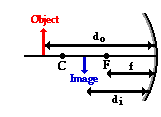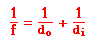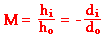Reflection and the Ray Model of Light - Lesson 3 - Concave Mirrors

# The Mirror Equation - Concave Mirrors

Ray diagrams can be used to determine the image location, size, orientation and type of image formed ofobjects when placed at a given location in front of a concave mirror. The use of these diagrams was demonstrated earlier in Lesson 3. Ray diagrams provide useful information about object-image relationships, yet fail to provide the information in a quantitative form. While a ray diagram may help one determine the approximate location and size of the image, it will not provide numerical information about image distance and object size. To obtain this type of numerical information, it is necessary to use the Mirror Equation and the Magnification Equation. The mirror equation expresses the quantitative relationship between the object distance (do), the image distance (di), and the focal length (f). The equation is stated as follows:The magnification equation relates the ratio of the image distance and object distance to the ratio of the image height (hi) and object height (ho). The magnification equation is stated as follows:These two equations can be combined to yield information about the image distance and image height if the object distance, object height, and focal length are known.

As a demonstration of the effectiveness of the mirror equation and magnification equation, consider the following example problem and its solution.

### Example Problem #1

A 4.00-cm tall light bulb is placed a distance of 45.7 cm from a concave mirror having a focal length of 15.2 cm. Determine the image distance and the image size.

Like all problems in physics, begin by the identification of the known information.

 ho = 4.0 cm do = 45.7 cm f = 15.2 cm

Next identify the unknown quantities that you wish to solve for.

 di = ??? hi = ???

To determine the image distance, the mirror equation must be used. The following lines represent the solution to the image distance; substitutions and algebraic steps are shown.

1/f = 1/do + 1/di

1/(15.2 cm) = 1/(45.7 cm) + 1/di

0.0658 cm-1 = 0.0219 cm-1 + 1/di

0.0439 cm-1 = 1/di

 di = 22.8 cm

The numerical values in the solution above were rounded when written down, yet un-rounded numbers were used in all calculations. The final answer is rounded to the third significant digit.

To determine the image height, the magnification equation is needed. Since three of the four quantities in the equation (disregarding the M) are known, the fourth quantity can be calculated. The solution is shown below.

hi/ho = - di/do

hi /(4.0 cm) = - (22.8 cm)/(45.7 cm)

hi = - (4.0 cm) • (22.8 cm)/(45.7 cm)

 hi = -1.99 cm

The negative values for image height indicate that the image is an inverted image. As is often the case in physics, a negative or positive sign in front of the numerical value for a physical quantity represents information about direction. In the case of the image height, a negative value always indicates an inverted image.

From the calculations in this problem it can be concluded that if a 4.00-cm tall object is placed 45.7 cm from a concave mirror having a focal length of 15.2 cm, then the image will be inverted, 1.99-cm tall and located 22.8 cm from the mirror. The results of this calculation agree with the principles discussed earlier in this lesson. In this case, the object is located beyond the center of curvature (which would be two focal lengths from the mirror), and the image is located between the center of curvature and the focal point. This falls into the category of Case 1 : The object is located beyond C.

Now lets try a second example problem:

### Example Problem #2

A 4.0-cm tall light bulb is placed a distance of 8.3 cm from a concave mirror having a focal length of 15.2 cm. (NOTE: this is the same object and the same mirror, only this time the object is placed closer to the mirror.) Determine the image distance and the image size.

Again, begin by the identification of the known information.

 ho = 4.0 cm do = 8.3 cm f = 15.2 cm

Next identify the unknown quantities that you wish to solve for.

 di = ??? hi = ???

To determine the image distance, the mirror equation will have to be used. The following lines represent the solution to the image distance; substitutions and algebraic steps are shown.

1/f = 1/do + 1/di

1/(15.2 cm) = 1/(8.3 cm) + 1/di

0.0658 cm-1 = 0.120 cm-1 + 1/di

-0.0547 cm-1 = 1/di

 di = -18.3 cm

The numerical values in the solution above were rounded when written down, yet un-rounded numbers were used in all calculations. The final answer is rounded to the third significant digit.

To determine the image height, the magnification equation is needed. Since three of the four quantities in the equation (disregarding the M) are known, the fourth quantity can be calculated. The solution is shown below.

hi/ho = - di/do

hi /(4.0 cm) = - (-18.2 cm)/(8.3 cm)

hi = - (4.0 cm) • (-18.2 cm)/(8.3 cm)

 hi = 8.8 cm

The negative value for image distance indicates that the image is a virtual image located behind the mirror. Again, a negative or positive sign in front of the numerical value for a physical quantity represents information about direction. In the case of the image distance, a negative value always means behind the mirror. Note also that the image height is a positive value, meaning an upright image. Any image that is upright and located behind the mirror is considered to be a virtual image.

From the calculations in the second example problem it can be concluded that if a 4.0-cm tall object is placed 8.3 cm from a concave mirror having a focal length of 15.2 cm, then the image will be magnified, upright, 8.8-cm tall and located 18.3 cm behind the mirror. The results of this calculation agree with the principles discussed earlier in this lesson. In this case, the object is located in front of the focal point (i.e., the object distance is less than the focal length), and the image is located behind the mirror. This falls into the category of Case 5: The object is located in front of F.

### The +/- Sign Conventions

The sign conventions for the given quantities in the mirror equation and magnification equations are as follows:

• f is + if the mirror is a concave mirror
• f is - if the mirror is a convex mirror
• di is + if the image is a real image and located on the object's side of the mirror.
• di is - if the image is a virtual image and located behind the mirror.
• hi is + if the image is an upright image (and therefore, also virtual)
• hi is - if the image an inverted image (and therefore, also real)

Like many mathematical problems in physics, the skill is only acquired through much personal practice. Perhaps you would like to take some time to try the problems in the Check Your Understanding section below.

### We Would Like to Suggest ...Why just read about it and when you could be interacting with it? Interact - that's exactly what you do when you use one of The Physics Classroom's Interactives. We would like to suggest that you combine the reading of this page with the use of our Optics Bench Interactive or our Name That Image Interactive. You can find this in the Physics Interactives section of our website. The Optics Bench Interactive provides the learner an interactive enivronment for exploring the formation of images by lenses and mirrors. The Name That Image Interactive provides learners with an intensive mental workout in recognizing the image characteristics for any given object location in front of a curved mirror.

### Check Your Understanding

1. Determine the image distance and image height for a 5.00-cm tall object placed 45.0 cm from a concave mirror having a focal length of 15.0 cm.

2. Determine the image distance and image height for a 5.00-cm tall object placed 30.0 cm from a concave mirror having a focal length of 15.0 cm.

3. Determine the image distance and image height for a 5.00-cm tall object placed 20.0 cm from a concave mirror having a focal length of 15.0 cm.

4. Determine the image distance and image height for a 5.00-cm tall object placed 10.0 cm from a concave mirror having a focal length of 15.0 cm.

5. A magnified, inverted image is located a distance of 32.0 cm from a concave mirror with a focal length of 12.0 cm. Determine the object distance and tell whether the image is real or virtual.

ZINGER: 6. An inverted image is magnified by 2 when the object is placed 22 cm in front of a concave mirror. Determine the image distance and the focal length of the mirror.

Next Section: